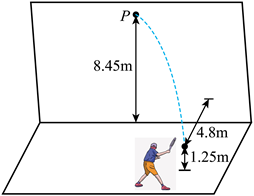$\text{A.}$ $v=5 \mathrm{~m} / \mathrm{s}$ $\text{B.}$ $v=3 \sqrt{2} \mathrm{~m} / \mathrm{s}$ $\text{C.}$ $d=3.6 \mathrm{~m}$ $\text{D.}$ $d=3.9 \mathrm{~m}$

BD

#### 解析：

$$v_{\text {0水平 }}=\sqrt{13^2-12^2} \mathrm{~m} / \mathrm{s}=5 \mathrm{~m} / \mathrm{s}$$